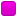October 05, 2023, 12:20:56 am

###AuthorTopic: tricky vectors question (lengthy)  (Read 3542 times) Tweet Share

0 Members and 1 Guest are viewing this topic.

#### peteypanda

• Fresh Poster
•• Posts: 1
• Respect: 0##### tricky vectors question (lengthy)
« on: April 29, 2020, 08:33:21 pm »
0
This is a real-life vectors question and I'm having trouble with part (ii) of this question. I have completed (i) A-C, but am completely stuck at part (ii) A-B. Pls help(i) Jack and Jim have decided to row across a river. They can row at a speed of 6.75 km/hr, and they are going to row due West. The current is flowing due South at 3.5 km/hr and there is a breeze blowing South-East at 3 km/hr.
a) Draw a vector diagram to represent this situation
b) Find the resultant velocity of the boat? (in relation to the East)
c)  If the river is 1.40 kilometres wide, then how much time does it take the boys to travel from shore to shore, in minutes? (Correct to 1 decimal place)
d)  What distance downstream, in metres, would the boat reach the opposite shore? (correct to the nearest whole number)

(ii) Jack and Jim had arranged to be picked up on the other side of the River. Unfortunately for the boys, the wind had a big change, 4 mins after they started to row. The breeze is now blowing due East at 4 km/hr.
a) Draw a vector diagram to represent this situation, using their starting point as the origin, to find their resultant vector position in metres in relation to the East, (correct to the nearest whole number), at 4 mins.  On the same diagram, assuming their boat speed and the river current speed is still the same, represent what is happening with vectors now that the breeze has changed both direction and speed. (4 marks)
b) Now once they reach the other side of the river, how far, and in which direction, do they have to walk to get to their pick up when they get to the opposite side? (4 marks)

#### RuiAce

• ATAR Notes Lecturer
• Honorary Moderator
• Great Wonder of ATAR Notes
••• Posts: 8814
• "All models are wrong, but some are useful."
• Respect: +2575##### Re: tricky vectors question (lengthy)
« Reply #1 on: May 13, 2020, 10:43:41 pm »
+2
Sorry for the significant delay! I've been spending the past few weeks heavily focused on university exams.

So firstly I feel that the question could be made clearer. There was a fair bit of guesswork that was needed. Less important is that I had to figure out what way the river was going, but that could be inferred after I saw that the current went south. More importantly, it wasn't really obvious to me that Jack and Jim were rowing from the east shore of the river. That was entirely a guess given that they were rowing due west.

I'm also not sure what the point of "in relation to the East" was. I suppose you could treat the easterly direction as the positive $y$-axis, and correspondingly the southerly direction as the positive $x$-axis. But I didn't see the point of that, so these numbers are just assuming the standard orientation (where the positive $y$-axis points in the northerly direction).

Nitty gritty details aside, the resultant velocity vector I attained in part(i), subpart b) was
\begin{align*}
\underset{\sim}{\dot{r}} &= \begin{pmatrix}-6.75\\ 0 \end{pmatrix} + \begin{pmatrix}0\\ -3.5\end{pmatrix} + \begin{pmatrix} 3\cos 45^\circ\\ -3\sin 45^\circ\end{pmatrix} \\
&= \begin{pmatrix} -6.75 + \frac{3}{\sqrt2}\\ -3.5 - \frac{3}{\sqrt2}\end{pmatrix}.
\end{align*}
(Or approximately $-4.63\underset\sim i -5.62 \underset\sim j$.)

Then you would've solved some equation similar to $\left( -6.75 + \frac{3}{\sqrt2} \right)t = -1.4$ for subpart c), and used the value for $t$ for d).

Now for part (ii), we essentially need to cater for the wind direction change at time $t=\frac1{15}$. (Carefully note the conversion from minutes to hours, because the speed was given in km/hr.) Because we're assuming that at that exact moment the wind changes (in particular to 4km/hr in the East direction), we have to first determine where the two are at time $t=\frac1{15}$. So we need the corresponding displacement vector to the original scenario first. Relative to the initial starting point (i.e. where they row), this is
$\underset{\sim}{r} = \begin{pmatrix} \left(-6.75 + \frac{3}{\sqrt2}\right)t \\ \left(-3.5 - \frac{3}{\sqrt2}\right)t\end{pmatrix}.$
When $t=\frac1{15}$,
$\underset{\sim}{r} \approx -0.308578644\underset \sim i-0.37475468 \underset\sim j$.

Then, after time $t=\frac{1}{15}$, we now have a new velocity vector. The velocity is now given by
\begin{align*}
\underset{\sim}{\dot{r}} &= \begin{pmatrix}-6.75\\ 0 \end{pmatrix} + \begin{pmatrix}0\\ -3.5\end{pmatrix} + \begin{pmatrix}4\\ 0\end{pmatrix}\\
&= \begin{pmatrix} -2.75\\ -3.5\end{pmatrix}.
\end{align*}
(Note that on your vector diagram, you should now have the vector of length 3.75 pointing SE, now having a length 4 pointing E.)

To simplify things out, let $T$ be the time elapsed from 4 minutes since everything began. This immediately implies that $T = t + \frac1{15}$, but we don't need this fact since we don't actually care about when exactly they land on the shore. We just care about where that point is.

But of course, they no longer need to travel a horizontal displacement of $-1.4$ now. Rather, they need to travel a horizontal displacement of $-1.4 - (-0.308578644) \approx -1.0914$. So you would be solving $-1.0914 = -2.75T$, and then computing $-3.5\times T$ for the vertical component.

At the very end, once again we have some ambiguity, because it's not made clear at all where exactly they were going to be picked up. So the only valid assumption would be that they were going to be picked up at where the boat was originally going to land. Therefore, you would compare your answer here to that of part (i) subpart d), to determine if they need to work north or south, and by how much.